# Excel COUNTIF and COUNTIFS with OR logic

The tutorial explains how to use Excel's COUNTIF and COUNTIFS functions to count cells with multiple OR conditions, e.g. if a cell contains X, Y or Z.

As everyone knows, Excel COUNTIF function is designed to count cells based on just one criterion while COUNTIFS evaluates multiple criteria with AND logic. But what if your task requires OR logic - when several conditions are provided, any one can match to be included in the count?

There are a few possible solutions to this task, and this tutorial will cover them all in full detail. The examples imply that you have a sound knowledge of the syntax and general uses of both functions. If not, you may want to begin with revising the basics:

Excel COUNTIF function - counts cells with one criteria.

Excel COUNTIFS function - counts cells with multiple AND criteria.

Now that everyone is on the same page, let's dive in:

## Count cells with OR conditions in Excel

This section covers the simplest scenario - counting cells that meet any (at least one) of the specified conditions.

### Formula 1. COUNTIF + COUNTIF

The easiest way to count cells that have one value or another (Countif a or b) is to write a regular COUNTIF formula to count each item individually, and then add the results:

COUNTIF(range, criterion1) + COUNTIF(range, criterion2)

As an example, let's find out how many cells in column A contain either "apples" or "bananas":

`=COUNTIF(A:A, "apples") + COUNTIF(A:A, "bananas")`

In real-life worksheets, it is a good practice to operate on ranges rather than entire columns for the formula to work faster. To spare the trouble of updating your formula every time the conditions change, type the items of interest in predefined cells, say F1 and G1, and reference those cells. For example:

`=COUNTIF(A2:A10, F1) + COUNTIF(A2:A10, G1)`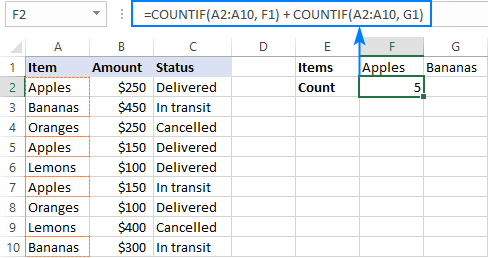This technique works fine for a couple of criteria, but adding three or more COUNTIF functions together would make the formula too cumbersome. In this case, you'd better stick with one of the following alternatives.

### Formula 2. COUNTIF with array constant

Here's a more compact version of the SUMIF with OR conditions formula in Excel:

SUM(COUNTIF(range, {criterion1, criterion2, criterion3, …}))

The formula is constructed in this way:

First, you package all the conditions in an array constant - individual items separated by commas and the array enclosed in curly braces like {"apples", "bananas', "lemons"}.

Then, you include the array constant in the criteria argument of a normal COUNTIF formula: COUNTIF(A2:A10, {"apples","bananas","lemons"})

Finally, warp the COUNTIF formula in the SUM function. It is necessary because COUNTIF will return 3 individual counts for "apples", "bananas" and "lemons", and you need to add those counts together.

Our complete formula goes as follows:

`=SUM(COUNTIF(A2:A10,{"apples","bananas","lemons"}))`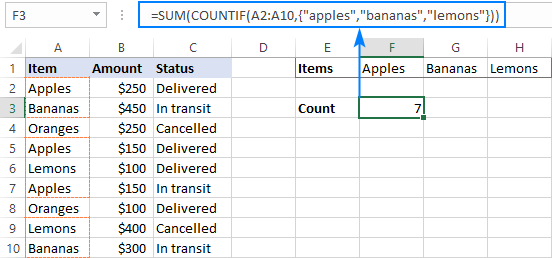If you'd rather supply your criteria as range references, you'll need to enter the formula with Ctrl + Shift + Enter to make it an array formula. For example:

`=SUM(COUNTIF(A2:A10,F1:H1))`

Please notice the curly braces in the screenshot below - it is the most evident indication of an array formula in Excel: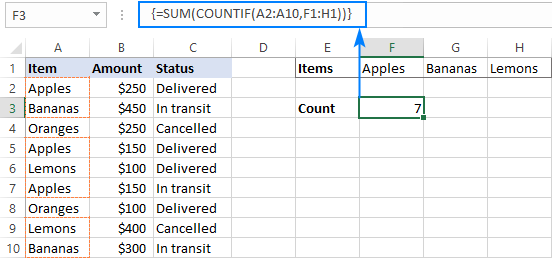### Formula 3. SUMPRODUCT

Another way to count cells with OR logic in Excel is to use the SUMPRODUCT function in this way:

SUMPRODUCT(1*(range ={criterion1, criterion2, criterion3, …}))

To better visualize the logic, this could also be written as:

SUMPRODUCT((range=criterion1) + (range=criterion2) + …)

The formula tests each cell in the range against each criterion and returns TRUE if the criterion is met, FALSE otherwise. As an intermediate result, you get a few arrays of TRUE and FALSE values (the number of arrays equals the number of your criteria). Then, the array elements in the same position are added together, i.e. the first elements in all the arrays, the second elements, and so on. The addition operation converts the logical values to numbers, so you end up with one array of 1's (one of the criteria matches) and 0's (none of the criteria matches). Because all the criteria are tested against the same cells, there is no way any other number could appear in the resulting array - only one initial array can have TRUE in a specific position, others will have FALSE. Finally, SUMPRODUCT adds up the elements of the resulting array, and you get the desired count.

The first formula works in a similar manner, with the difference that it returns one 2-dimentional array of TRUE and FALSE values, which you multiply by 1 to convert the logical values to 1 and 0, respectively.

Applied to our sample data set, the formulas take the following shape:

`=SUMPRODUCT(1*(A2:A10={"apples","bananas","lemons"}))`

Or

`=SUMPRODUCT((A2:A10="apples") + (A2:A10="bananas") + (A2:A10="lemons"))`

Replace the hardcoded array constant with a range reference, and you will get even a more elegant solution:

`=SUMPRODUCT(1*( A2:A10=F1:H1))`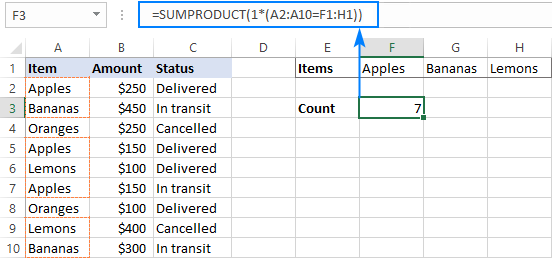Note. The SUMPRODUCT function is slower than COUNTIF, which is why this formula is best to be used on relatively small data sets.

## Count cells with OR as well as AND logic

When working with large data sets that have multi-level and cross-level relations between elements, chances are that you will need to count cells with OR and AND conditions at a time.

As an example, let's get a count of "apples", "bananas" and "lemons" that are "delivered". How do we do that? For starters, let's translate our conditions into Excel's language:

• Column A: "apples" or "bananas" or "lemons"
• Column C: "delivered"

Looking from another angle, we need to count rows with "apples and delivered" OR "bananas and delivered" OR "lemons and delivered". Put this way, the task boils down to counting cells with 3 OR conditions - exactly what we did in the previous section! The only difference is that you'll utilize COUNTIFS instead of COUNTIF to evaluate the AND criterion within each OR condition.

### Formula 1. COUNTIFS + COUNTIFS

It is the longest formula, which is the easiest to write :)

`=COUNTIFS(A2:A10, "apples", C2:C10, "delivered") + COUNTIFS(A2:A10, "bananas", C2:C10, "delivered")) + COUNTIFS(A2:A10, "lemons", C2:C10, "delivered"))`

The screenshot below shows the same formula with cells references:

`=COUNTIFS(A2:A10, K1, C2:C10, K2) + COUNTIFS(A2:A10, L1, C2:C10, K2) + COUNTIFS(A2:A10, M1,C2:C10, K2)`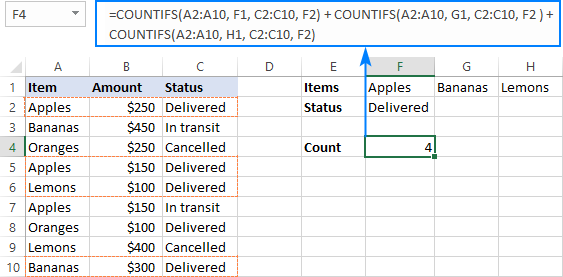### Formula 2. COUNTIFS with array constant

A more compact COUNTIFS formula with AND/OR logic can be created by packaging OR criteria in an array constant:

`=SUM(COUNTIFS(A2:A10, {"apples","bananas","lemons"}, C2:C10, "delivered"))`

When using a range reference for the criteria, you need an array formula, completed by pressing Ctrl + Shift + Enter:

`=SUM(COUNTIFS(A2:A10,F1:H1,C2:C10,F2))`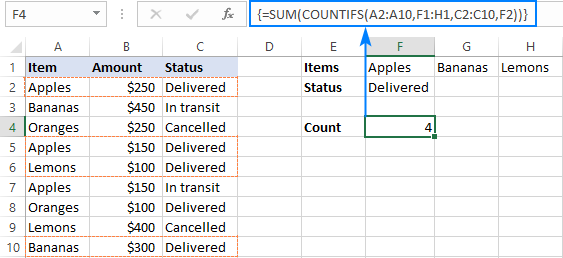Tip. If needed, you are free to use wildcards in the criteria of any formulas discussed above. For example, to count all sorts of bananas such as "green bananas" or "goldfinger bananas" you can use this formula:

`=SUM(COUNTIFS(A2:A10, {"apples","*bananas*","lemons"}, C2:C10, "delivered"))`

In a similar manner, you can build a formula to count cells based on other criteria types. For example, to get a count of "apples" or "bananas" or "lemons" that are "delivered" and the amount is greater than 200, add one more criteria range/criteria pair to COUNTIFS:

`=SUM(COUNTIFS(A2:A10, {"apples","*bananas*","lemons"}, C2:C10, "delivered", B2:B10, ">200"))`

Or, use this array formula (entered via Ctrl + Shift + Enter):

`=SUM(COUNTIFS(A2:A10,F1:H1,C2:C10,F2, B2:B10, ">"&F3))`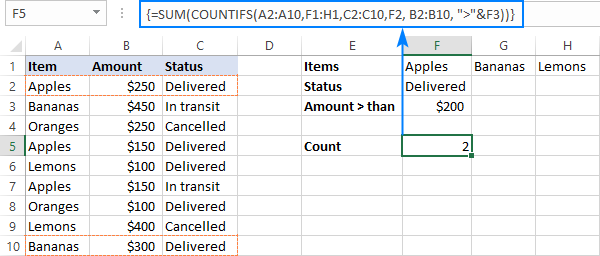## Count cells with multiple OR conditions

In the previous example, you have learned how to test one set of OR conditions. But what if you have two or more sets and you are looking to get a total of all possible OR relations?

Depending on how many conditions you need to handle, you can use either COUNTIFS with an array constant or SUMPRODUCT with ISNUMBER MATCH. The former is relatively easy to build, but it is limited to only 2 sets of OR conditions. The latter can evaluate any number of conditions (a reasonable number, of course, given Excel's limit to 255 arguments and 8192 characters to the total formula length), but it may take some effort to grasp the formula's logic.

### Count cells with 2 sets of OR conditions

When dealing with only two sets of OR criteria, just add one more array constant to the COUNTIFS formula discussed above.

For the formula to work, one minute but critical change is needed: use a horizontal array (elements separated by commas) for one criteria set and vertical array (elements separated by semicolons) for the other. This tells Excel to "pair" or "cross-calculate" the elements in the two arrays, and return a two-dimensional array of the results.

As an example, let's count "apples", "bananas" or "lemons" that are either "delivered" or "in transit":

`=SUM(COUNTIFS(A2:A10, {"apples", "bananas", "lemons"}, B2:B10, {"delivered"; "in transit"}))`

Please note the semicolon in the second array constant: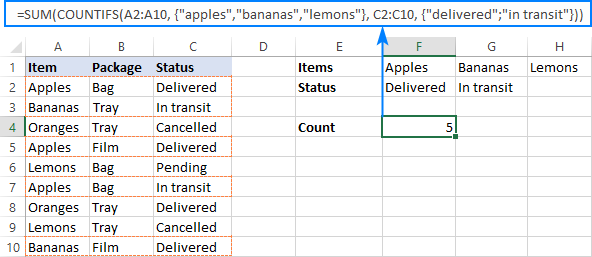Because Excel is a 2-dimentional program, it is not possible to construct a 3-dimentional or 4-dimentuional array, and therefore this formula only works for two sets of OR criteria. To count with more criteria, you will have to switch to a more complex SUMPRODUCT formula explained in the next example.

### Count cells with multiple sets of OR conditions

To count cells with more than two sets of OR criteria, use the SUMPRODUCT function together with ISNUMBER MATCH.

For example, let's get a count of "apples", "bananas" or "lemons" that are either "delivered" or "in transit" and are packaged in either "bag" or "tray":

```=SUMPRODUCT(ISNUMBER(MATCH(A2:A10,{"apples","bananas","lemons"},0))* ISNUMBER(MATCH(B2:B10,{"bag","tray"},0))* ISNUMBER(MATCH(C2:C10,{"delivered","in transit"},0))) ```

In the heart of the formula, the MATCH function checks the criteria by comparing each cell in the specified range with the corresponding array constant. If the match is found, it returns a relative position of the value if the array, N/A otherwise. ISNUMBER converts these values to TRUE and FALSE, which equate to 1 and 0, respectively. SUMPRODUCT takes it from there, and multiplies the arrays' elements. Because multiplying by zero gives zero, only the cells that have 1 in all the arrays survive and get summed.

Th screenshot below shows the result: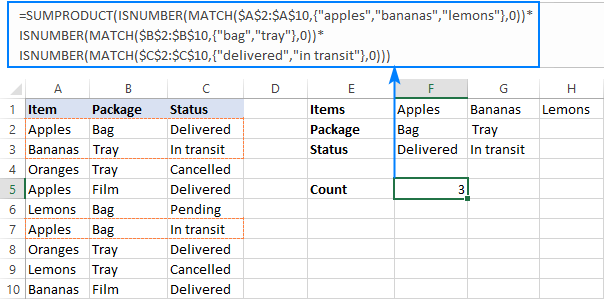This is how you use the COUNTIF and COUNTIFS functions in Excel to count cells with multiple AND as well as OR conditions. To have a closer look at the formulas discussed in this tutorial, you are welcome to download our sample workbook below. I thank you for reading and hope to see you on our blog next week!

## Practice workbook

Excel COUNTIF with OR conditions - examples (.xlsx file)

## You may also be interested in

1. I have a spreadsheet for degree summaries. For courses with prereqs I want it the add the prereq courses only if students have an A, B, or C. A couple courses have four prereqs. Currently, it's counting if a prereq is not blank, and then I have to double-check the grade, which can lead to errors. Is this possible?

2. I have a spreadsheet where I am using the following countifs formulas: =COUNTIFS(LOG!\$H\$2:\$H\$1249,"*Initial*",LOG!\$K\$2:\$K\$1249,"Anderson*")
I also need to add in one more item to consider: how may of those were received in a certain month. How would I do that?

3. Hi,

How can I use the Countif Function in an Array ? Say I have a 2-column data Column A has the days , viz Wed, Mon, Tue, Sat, Thu, Fri, Tue, Mon, Sat, ..... and Column B has a corresponding positive or negative number. I want to find out how many positive numbers are there in Column B when I select the Column A filter = "Tue".

Thanks

• To calculate the number of values for the two conditions, use the COUNTIFS function. All the information you need is in the article above. Here is an example of a formula:

=COUNTIFS(A1:A10,"Tue",B1:B10,">0")

• Thanks for the input. But this formula would be on a case to case basis. But when I am selecting a " Tue" or a "Wed" from the dropdown filter in Column A or multi select "Tue" &"Wed", I would like to find out the no. of counts of positive numbers in Column B for those Days which have been selected via the Drop Down filter of Column A.

Regards

• Hi! The filter in the column has no effect on the results of the formula calculation. You need to add OR logic as described in the article above. For example,

=COUNTIFS(A1:A10,"Tue",B1:B10,">0") + COUNTIFS(A1:A10,"Wed",B1:B10,">0")

• Actually , I am looking for a formula that will count the positive numbers based on the filter of Column A. It has to be an array formula.

4. Hello! Thank you for all the tutorials. They are ALL very helpful.

You might have covered this before, but I could not find it.

How can I count if more than one cell in a range contains one criterion (Yes)? I need to count how many people selected more than one race. (6 cells, how many are marked 'Yes')

Once again, thank you!
- Dánae

• Hi!
Your description does not exactly understand the question. If the recommendations in the article above don't work for you, try these: Count unique values with criteria. Or explain in more detail.

5. "Hello,
I need help with a task in a spreadsheet that involves searching a table for entries based on several criteria and counting them. Specifically, I need to find entries that meet the following conditions:

Column G must equal 'No' AND
Column O must be less than or equal to a date specified in cell C22 AND
Columns U, AK, and AL must either be equal to 0 OR greater than the date in cell C22

I would greatly appreciate any assistance or guidance on how to accomplish this task using Excel. Thank you in advance for your help!"

6. Hi ,

Let's say I have 2 columns Column A - 1-20 and Column B - 31 - 50. How can I count the unique entries when column A is > 10 or Column B is > 40. Kindly help.

7. Hi Sir,

I am creating schedules for our staff. Using below data and trying to count the actual working employees total and ignore employees those are on training and days off. I am using this formula but its not working . Please help me
=SUMIF(\$D\$6:\$D\$14,"TRAINING",F6:F14) + SUMIF(F6:F14,"D/O",F6:F14)
D E F G H
LEADS EMPL # Sun Mon Tue Wed Thu Fri Sat
1 TRAINING D/O D/O 05:45 - 14:15 05:45 - 14:15 05:45 - 14:15 05:45 - 14:15 05:45 - 14:15
2 ANNY 05:45 - 14:15 05:45 - 14:15 D/O D/O 05:45 - 14:15 05:45 - 14:15 05:45 - 14:15
3 PERRI 05:45 - 14:15 05:45 - 14:15 05:45 - 14:15 05:45 - 14:15 D/O D/O 05:45 - 14:15
4 JAM D/O 05:45 - 14:15 05:45 - 14:15 05:45 - 14:15 05:45 - 14:15 05:45 - 14:15 D/O
5 KLM 05:45 - 14:15 D/O D/O 05:45 - 14:15 05:45 - 14:15 05:45 - 14:15 05:45 - 14:15
6 BOBBY 05:45 - 14:15 05:45 - 14:15 05:45 - 14:15 D/O D/O 05:45 - 14:15 05:45 - 14:15
7 TRAINING 05:45 - 14:15 05:45 - 14:15 05:45 - 14:15 05:45 - 14:15 05:45 - 14:15 D/O D/O
8 TRAINING D/O D/O 05:45 - 14:15 05:45 - 14:15 05:45 - 14:15 05:45 - 14:15 05:45 - 14:15
9 TRAINING 05:45 - 14:15 05:45 - 14:15 D/O D/O 05:45 - 14:15 05:45 - 14:15 05:45 - 14:15
Daily Total 6 6 6 6 7 7 7
Actual Daily 4 4 0 0 0 0 0
Variance +(-) 2

• Hi!
Use the COUNTIF function instead of the SUMIF function as described in the article above.

• Hi sir,, thanks so much for yo class it's very interesting I've added something new..
Can you let me know if there is an excell formal that can colour the cell with a certain commands??

• I mean that,, is there a formula that can help me to make a cell coloured??

8. Hi
My current issue is i have to sheets lets say
Sheet1 is where the formula is so
Countif(sheet2A:A;\$C\$3) this formula will be in cell C5 and the C6,C7, C8

What i need is to move the auto fill series to move ranges on sheet2 to be filled as the formula on C6 to query B:B, C7 to query C:C and so on

Can you help me with that please

• Hi!
Copying the formula down may change the row numbers, but not the column numbers. Autofill is not working the way you want.
You can dynamically change cell addresses in formulas using the INDIRECT function.

9. What do I do if I need to do count cells with multiple sets of OR conditions, but one of or conditions is not match, but rather a countif (ie >0)? Thanks!

• Hi!
Have you tried the ways described in this blog post? If they don’t work for you, then please describe your task in detail, I’ll try to suggest a solution.

10. Hi, I'm trying to assemble multiple AND/OR logic. The first group I can solve with SUM(COUNTIFS, but getting stuck after the OR logic

Count cells if:
column F is one of: "*Scope*","*Component*","*Vendor*" AND column E = A2 (let's call this group1)
OR
column F = "*Decommission*" AND column I "*Production*" AND column E = A2 (let's call this group2)

Final result should be total cell count of group1 + group2
(wildcards intentional)
Thank you

• note in the second group column I doesn't equal "*Production*" - looks like my symbols were removed

• Hi!
Have you tried the ways described in this blog post? It covers your case completely.
Here is an example formula

=COUNTIFS(F1:F10,"*Scope*",E1:E10,\$A\$2)+COUNTIFS(F1:F10,"*Component*",E1:E10,\$A\$2)+COUNTIFS(F1:F10,"*Vendor*",E1:E10,\$A\$2)

11. I have excel sheet for result analysis range H7:ET600. I have to count "AB" from some columns {e.g. J, W, AJ, AW, BJ, BV, CC, CO, DB, DO, EB, EP columns } only. I can't select range because columns other than {J, W, AJ, AW, BJ, BV, CC, CO, DB, DO, EB, EP} these one also have "AB" entry. how I can count it?

• Hi!
If I understand your task correctly, the example following formula should work for you:

=SUM(--(A1:A10="AB"),--(C1:C10="AB"),--(E1:E10="AB"))

Specify each column individually.

12. How to count the occurrence of each number (range from 1 to 14) in a cell with a number pattern 1/12/5/1/13/10 or 10/11/9 or 8/5 ?

13. Good day
I'm trying to automate a count on a per class basis and a age.

my current formula is this:

=SUM(COUNTIFS('PC Spec CPT'!\$A\$1:\$L\$171, "*CLASS1*", 'PC Spec CPT'!\$A\$1:\$L\$171, {"5 years","6 years","7 years","8 years","9 years","10 years"}))

This is returning a value of 0
but if I do the counts separately I get the correct values. so "count class1", gives 39 and count (the different years) gives a value of 151 (as its searching the whole data set, but I only need it to count for class1 (Which is actually from A3 to L41) but I want to future proof for if I add or remove from the set

So the count cant be 0

• I think i may have figured it out...
=SUM(COUNTIFS('PC Spec CPT'!\$B\$3:\$B\$171, "*CLASS1*",'PC Spec CPT'!\$J\$3:\$J\$171,{"5 years","6 years","7 years","8 years","9 years","10 years"}))

Appears to give the correct result... I just needed to isolate the columns for the 2 criteria instead of doing the whole data set

• Hi!
The COUNTIFS formula counts the rows where all the criteria are met once. But at the same time, it cannot work with a range (\$A\$1:\$L\$171), but only with a data column (\$A\$1:\$A\$171).

14. Awesome! Thanks a lot for all clarifications in here!

15. Can you help....?

I have a spreadsheet with data collected from an electronically completed survey. Some of the columns are free text entry from the form. I want to summarise the submissions and categorise according to job role. However, there are a huge number of terms used for example for doctors....."registrar", "SHO", "consultant" "consultant anaesthetist", "anaesthetist", "FY2", "clinical fellow" etc. I am trying to use SUM and COUNTIFS to give me the number of forms that were submitted for a group of staff in one month. However, I am getting entries double counted as if the free text includes "consultant anaesthetist" then it is being counted twice as I want to ensure that entries that have only described the doctor as a consultant or anaesthetist are counted....

This is my formula I have named ranges Date and Recipient, H5 and I5 are cell references to specific dates.

=SUM(COUNTIFS(Date,">="&H\$5,Date,"<"&I\$5,Recipient,{"*Doctor*","*anaesthetist*","*surgeon*","*consultant*","*SAS*","*fellow*","*sho*","*reg*","*CT1*","*CT2*","*CT3*","*ST1*","*ST2*","*ST3*","*ST4*","*ST5*","*ST6*","*ST7*","*FY1*","*FY2*","associate specialist"}))

• Hello!
To avoid double counting, in a separate column, write something like this SEARCH formula with all the words you need. Then use that column to count in the COUNTIFS formula.

=--(SUM(--ISNUMBER(SEARCH({"Doctor","anaesthetist"},A2)))>0)